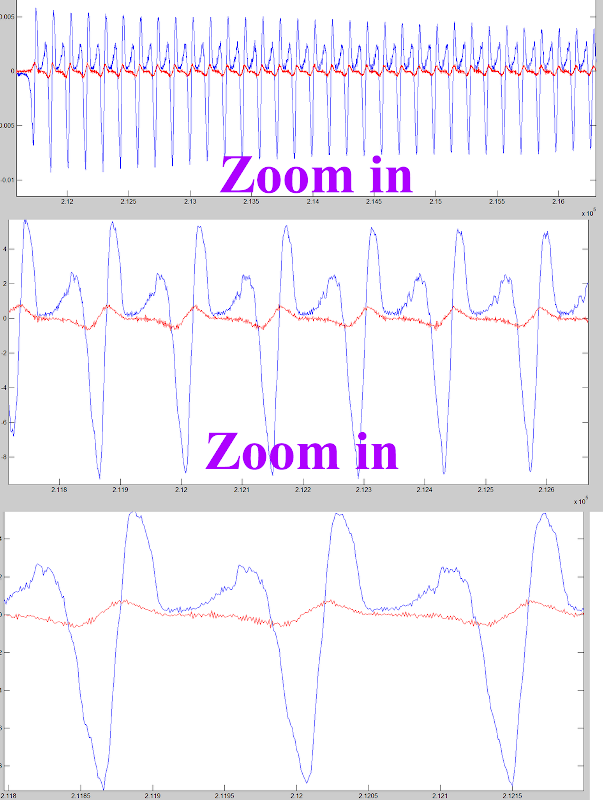# Subtracting sensors output that have mutual inductnace between them

Background: The software that i use to anaylze my signals is matlab. I have two audio signals that were recorded using two magnetic sensors. Lets call one sensor A and the other one B. A and B have mutual inductance between them.

While sensors A and B are working, I would like to subtract the information that was received in sensor A due to mutual inductance from sensor B.

I tried to subtract signal B from signal A, by simply writing in matlab (A-B), but it gives me a wierd answer. I think that it derives from a shift that I have in phase. The beginning recording time of the two tracks is the same, and therefore I think that it is not a time delay.

I would like to know how to do this subtraction process in theory, and if anyone have any tips how to implement it easily in matlab I would like to know.

I will really appreciate any help.

Below I attached pictures of the signals graphs. In the first pic you can see the signals that are received from sensor A and sensor B. In the second picture, I plotted sensor A in red and sensor B in blue, in the range of 2.12:2.16 and zoomed in even more.• So from your plots, it appears that there isn't much crosstalk from source 1 to source 2, only from 2 to 1. Is your overlay on the image incorrect then ("This has to be subtracted from source 2")? Seems like you're trying to remove source 2 from 1, right? – Jason R Oct 28 '11 at 16:28
• That's correct. – user1017064 Oct 28 '11 at 16:50
• to confirm the phase shift idea, plot both waveforms up close, only including from index 2.3 to 2.4 or something, so you can see individual wave in both channels – endolith Oct 28 '11 at 22:57

That looks like a straight forward cross-talk problem. You cannot simply subtract out the signal directly since magnetic coupling is different for every frequency (in terms of amplitude and phase).

Let's say you have two audio signals xa(t) and xb(t) and two sensor signals ya(t) and yb(t). Since there is coupling between the sensors you will get cross talk and we can write in the frequency domain

Ya(w) = Haa(w)*xa(w) + Hba(w)*xb(w)
Yb(w) = Hab(w)*xa(w) + Hbb(w)*xb(w)


where Hxy(w) is the transfer function from signal "x" to sensor signal "y". The 4 transfer functions form a 2x2 matrix and in order to fully recover the original signals you need to invert the matrix and apply the inverted matrix transfer functions to your received sensor signals.

Since your cross talk is small, you can simply measure the transfer function Hba(w) directly and subtract it out as follows: Measure the transfer function from signal A to sensor B when signal B is 0. Create a filter from this transfer function (FIR or IIR, depending on it's shape). Now you can measure and subtract a filtered version from sensor signal A from sensor signal B:

yb(t)' = yb(t)-hab(t)**ya(t)


where hab(t) is the impulse response of your cross talk filter and ** the convolution operator.

The cross talk filter represents the amplitude and phase shift as a function of frequency of your specific sensor coupling and it ensures that the correct signal gets subtracted.

• Hilmar, I am new to matlab and I encountered some difficulties when trying to implement your answer. I will be greatful for any assistance from everybody. First thing I did was to record signal A when sensor B is 0. Now, which arguments shall I insert in filter when calling the function "filter"? If you need any visualization of the signal you can see it in the above question. Thanks again. – user1017064 Dec 1 '11 at 22:04

This sounds like blind source separation. In general, you can't unmix things after they've been mixed. If you have two different recordings of two sources with some of each source in each recording, you can sometimes use independent component analysis to separate them.

I have a Python example here. There's also FastICA for MATLAB. If they are audio signals, being picked up magnetically, there's probably no appreciable delay between them. ICA works well in this case.

• The FastICA for Matlab did not help me to make the subtraction between the two signals. The result is just not good enough. In my comment above I attached a link to a photo which can explain the situation that I am facing: bit.ly/rXmVgH . By the way thank you very much for your help. – user1017064 Oct 28 '11 at 14:40